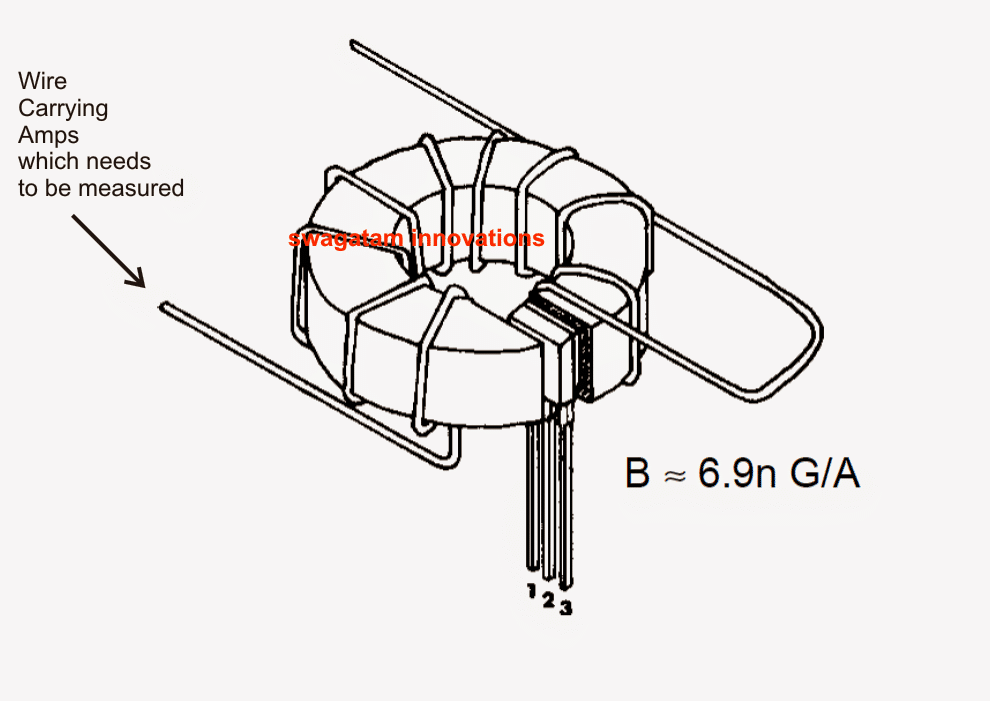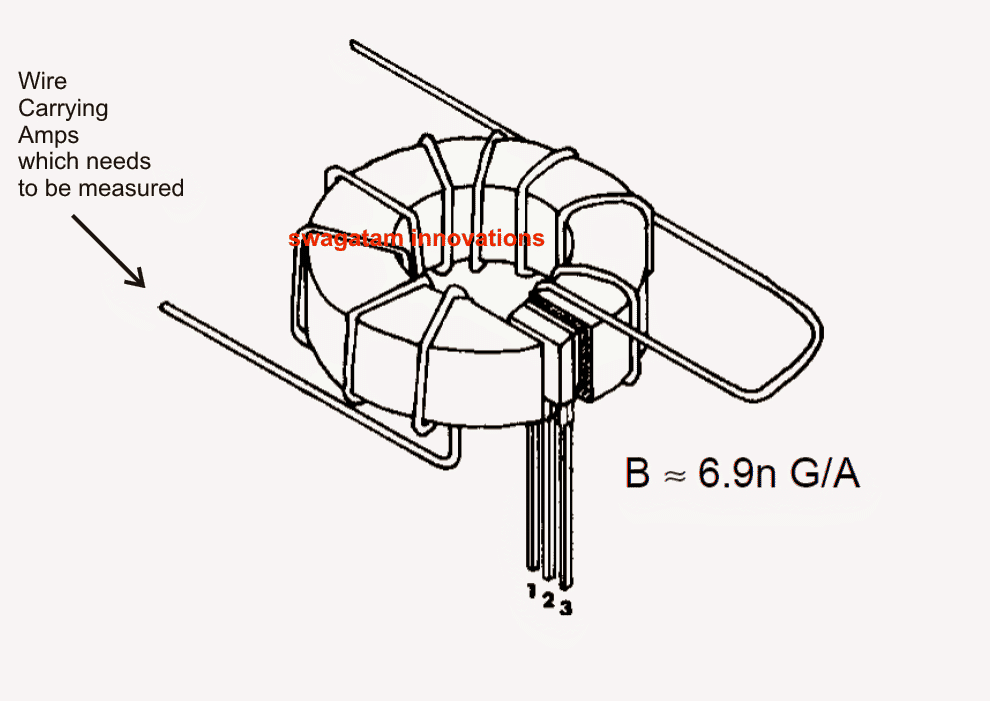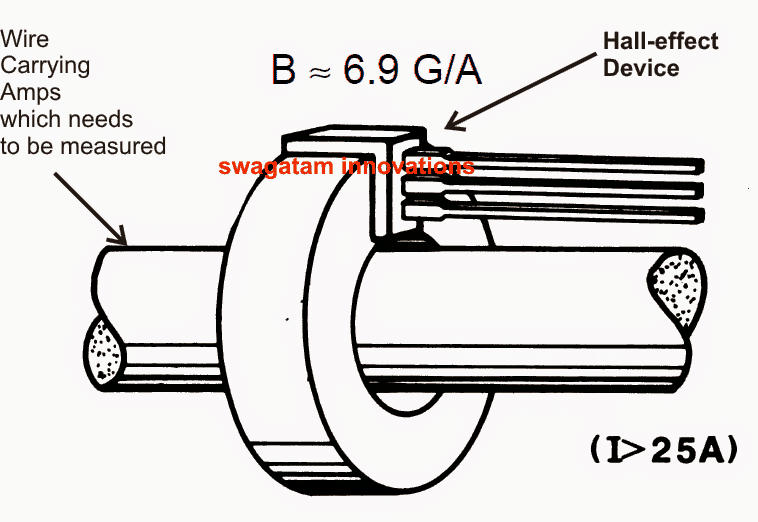Home » Electronic Sensors » Non-Contact Current Sensor Circuit Using Hall-Effect ICNon-Contact Current Sensor Circuit Using Hall-Effect IC

In this article we learn about a simple non-contact current sensor circuit using a hall effect sensor IC.

Contents

Why Hall Effect Sensor

When it comes to sensing current (Amps) linear Hall-effect devices are the best and the most accurate.

These devices can sense and measure current right from a few amps to many thousands. Moreover it allows the measurements to be done externally without necessitating a physical contact with the conductor.

When current passes through a conductor, typically a free-space magnetic field of around 6.9 gauss per ampere is generated.

This implies, in order to get a valid output from the Hall-effect device it needs to be configured within the range of the above field.

For conductors with low currents this means the device needs to be configured inside specially designed arrangements for enhancing the range and the sensing capabilities of the sensor.

However for conductor carrying high magnitudes of current, any special arrangement may not be required and the linear Hall-effect device would be capable of sensing and measuring the amps directly by positioning itself within a gapped torroid.

Calculating Magnetic Flux

The magnetic flux density over the device may be formulated as under:

B = I/4(pi)r, or I = 4(pi)rB

where,
B = field strength in Gauss
I = current in Amperes
r = distance from the center of the conductor to the positioned device in inches.

It may be noted that a Hall-effect element will produce the most optimal response when it's positioned perpendicular to a magnetic field. The reason being, reduced generation of cosine of the angle compared to angled fields at 90 degrees.

Non-contact measurement of current (low) Using a Coil and a Hall-effect device

As discussed above, when lower currents are involved measuring it through a coil becomes useful since the coil helps to concentrate the flux density and hence the sensitivity.

Enforcing Device-to-Coil Gap

By enforcing a device-to-coil air gap of 0.060" the effective magnetic flux density achieved becomes:

B = 6.9nI or n = B/6.9I

where n = number of turns of  coil.

As an example, for visualizing 400 gauss at 12 amperes, the above formula may be used as:

n = 400/83 = 5 turns

A conductor carrying lower magnitudes of current, typically below 1 gauss become difficult to sense due to the presence of inherent interference normally accompanied with solid-state devices and linear amplifier circuits.

The wide-band noise emitted at the output of the device being typically 400uV RMS, resulting an error of about 32mA, that could be significantly large.

In order to identify and measure low currents correctly, an arrangement shown below is utilized wherein the conductor is wrapped around a toroidal core a few number of times (n), giving the following equation:

B = 6.9nI

where n is the number of turns

The method allows low current magnetic fields to be enhanced sufficiently for providing the Hall-effect device an error free data for the subsequent conversion in volts.Non-contact measurement of current (high) Using a Toroid  and a Hall-effect device

In cases where the current through the conductor may be high (around 100 amps), a Hall-effect device may be directly used via a spit-section toroid for measuring the magnitudes in question.

As can be seen the figure below, the Hall-effect is placed between the split or the gap of the toroid while the conductor carrying the current passes through the torroid ring.

The magnetic field generated around the conductor is concentrated within the torroid and is detected by the Hall device for the required conversions at the output.The equivalent conversions made by the Hall-effect can be directly read by appropriately connecting its leads to a digital multimeter set at mV DC range.

The supply lead of the Hall-effect IC should be connected to a DC source as per its specifications.

Courtesy:

allegromicro.com/~/media/Files/Technical-Documents/an27702-Linear-Hall-Effect-Sensor-ICs.ashx

SHARING IS CARING!